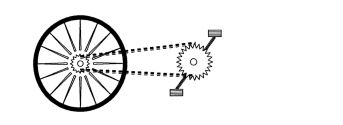# A cyclist moves with your bike straightA bike moves at a rate of $\si{18\ \kilo\meter/\hour}$. The rear tire has a diameter of $\si{70\ \centi\meter}$ and has a toothwheel of diameter $\si{7\ \centi\meter}$. The sprocket (the gear that's turned by the pedals) has diameter $\si{20\ \centi\meter}$. Both gears are connected by the chain and there is no slippage between the chain and either gear. Under these conditions, how many times do the pedals revolve per minute?

In this matter, assume $\pi = 3$.

×

Problem Loading...

Note Loading...

Set Loading...